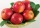# Greatest common divisor (GCD) + divisibility - math problems

#### Number of problems found: 35

• Lcm = 22 + gcdThe least common multiple of two numbers is 22 more than their greatest common divisor. Find these numbers.
• Coloured numbersMussel wrote four different natural numbers with coloured markers: red, blue, green and yellow. When the red number divides by blue, it gets the green number as an incomplete proportion, and yellow represents the remainder after this division. When it div
• PegsFrom two sticks 240 cm and 210 cm long, it is necessary to cut the longest possible pegs for flowers so that no residues remain. How many pegs will it be?
• Children's homeThe children's home received a gift to Nicholas of 54 oranges, 81 chocolate figurines, and 135 apples. Every child received the same gift and nothing was left. a) How many packages could be prepared? b) what did the children find in the package?
• Common divisorsFind all common divisors of numbers 30 and 45.
• Length of a stringWhat is the smallest length of a string that we can cut into 18 equal parts and even 27 equal parts (in decimeters)?
• Seven numbersWrite seven 4-digit numbers that are divisible by 3 and at the same time by 4.
• Reminder and quotientThere are given the number C = 281, D = 201. Find the highest natural number S so that the C:S, D:S are with the remainder of 1,
• Four poplarsFour poplars are growing along the way. The distances between them are 35 m, 14 m, and 91 m. At least how many poplars need to be dropped to create the same spacing between the trees? How many meters will it be?
• Bricks pyramidHow many 50cm x 32cm x 30cm brick needed to built a 272m x 272m x 278m pyramid?
• Trees in alleyThere are four trees in the alley between which the distances are 35m, 15m and 95m. Trees must be laid in the spaces so that the distance is the same and the maximum. How many trees will they put in and what will be the distance between them?
• MO C–I–1 2018An unknown number is divisible by just four numbers from the set {6, 15, 20, 21, 70}. Determine which ones.
• Three friendsThree friends had balls in ratio 2: 7: 4 at the start of the game. Could they have the same number of balls at the end of the game? Write 0, if not, or write the minimum number of balls they had together.
• GroupsIn the 6th class there are 60 girls and 72 boys. We want to divide them into groups so that the number of girls and boys is the same. How many groups can you create? How many girls will be in the group?
• Gardens colonyGardens colony with dimensions of 180 m and 300 m are to be completely divided into the same large squares of the highest area. Calculate how many such squares can be obtained and determine the length of the square side.
• Paving - jointsWe are paving with rectangular pavement 18 cm × 24 cm was placed side by side in height in a row and in the second row in width etc. How many times will the joints meet at a distance 10 m?
• GardenThe garden has the shape of a rectangle measuring 19m20cm and 21m60cm. Mr. Novák will fence it. It wants the distance between adjacent pillars to be at least two meters and a maximum of three meters. He would also like the distances between the adjacent p
• LCD 2The least common denominator of 2/5, 1/2, and 3/41st blade 2,5 m, 2nd blade. .1.75 m. How many same long pieces of this two blades can be do the biggest? How long is one piece?The room has dimensions of 12 meters and 5.6 meters. Determine the number of square tiles and their largest dimension to exactly cover the floor.